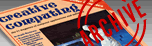`CREATIVE COMPUTING VOL. 9, NO. 6 / JUNE 1983 / PAGE 280`

 Rabbits and foxes: biological population cycles. (computer simulation) C.J.G. Raw. Rabbits and Foxes: Biological Population Cycles A very simple model of population oscillations in host-parasite or preypredator systems was developed by Lotka and Volterra in the 1920s, and is still useful today. A good reference to the mathematical background is G. Murdie's article on "Population Models' (chapter 8 of J.G. Andrews and R.R. McLone Mathematical Modelling, Butterworths, Inc., Boston, 1976). A very simple program (in Applesoft Basic) for demonstrating this model is presented here. It illustrates how very elementary programming may sometimes be used in dealing with sophisticated scientific ideas. The Lotka-Volterra model may be visualized as follows. I am using the rabbits-and-foxes prey-predator system as an example. A + X 2X X + Y 2Y Y D Here, A represents the (constant) fodd supply of the rabbits whose population (X) increases in the first step. The foxes prey on the rabbits to increase the fox population (Y) in step two. Finally, when the rabbit population has been reduced sufficiently, and the foxes (which are assumed to eat only rabbits) have become too numerous, the foxes begin to die off in step three for lack of food. This makes it possible for the rabbit population to begin increasing again via step one. The whole population cycles, then repeats itself. Although this model is somewhat simplified (we ignore the effect of weather on the rabbits' food supply, and we assume foxes live only on rabbits), it is still widely used as a basis for the study of fluctuating populations in prey-predator or host-parasite systems. The Lotka-Volterra differential equations express the time rate of increase of the populations X and Y (rabbits and foxes, respectively). It is not the purpose here to explain differential equations but I list them below for the calculus experts: (DX/DT) = (K1 - K2*Y)*X (DY/DT) = (K2*X - K3)*Y In these equations, K1 represents the intrinsic rate of increase of rabbits which is diminished in the first equation by a term proportional to the number of foxes (K2*Y). DK represents the increase in rabbit population in the very small time interval DT, with a similar interpreatation of DY. In the second equation above, the rate of increase of foxes is proportional to the rabbit population (K2*X) diminished by the death rate (K3) of foxes. Both rates are proportional overall to the parent populations (X and Y themselves). Note that in the absence of foxes, Y=0, the rabbit population grows exponentially: DX/DT = K1*X which integrates to (again for the calculus experts) X = X0 *EXP(K1 ) where X0 is the original rabbit population (at time T=0). The two Lotka-Volterra equations are solved in the general by computing the increments (DX and DY) for a very small time interval, DT = .0001, and adding these to the starting values of X and Y. This is repeated a very large number of times to compute how the populations of rabbits (X) and foxes (Y) change as time goes on. The program listed here plots the population of foxes (Y) versus rabbits (X), using the high-resolution page 1 graphics screen of the Apple II computer. The initial values of the populations X and Y, and appropriate positive values of the rate coefficients (K1, K2, and K3), are entered by the program user. Note that the populations should be scaled to fit the resolution along the axes. For simplicity, I have assumed that the X population could vary from 1 to 279, and the Y population from 1 to 159. Notice, also, that a steady state is possible. This occurs when the populations do not change with time, i.e. DX/DT = 0 and DY/DT = 0. From the Lotka-Volterra equations, it follows that the steady state populations are X = K3/K2 and Y = K1/K2. The values of the K1, K2, and K3 should be chosen so that the steady state populations lie near the center of the screen. The rate coefficients and initial populations are dispyayed in the four lines of text at the bottom of the high-resolution page 1 screen. I used a Silentype thermal printer to display both the listing and a typical RUN. It might be interesting for the reader to try other values for the initial populations and rate coefficients, just to see what happens. My own interest in this model arose from its application to oscillations in the concentrations of certain intermediate species in oscillatory chemical reactions. The model requires considerable extension to account satisfactorily for such chemical reactions, however. For the reader interested in such extensions to the chemical, biological and social scienes, an excellent reference is Nicolis and Prigogine's "Self-Organization in Non-Equilibrium Systems' (Wiley-Interscience, New York, 1977). Professor Prigogine won the Nobel Prize in chemistry for this work a few years ago.